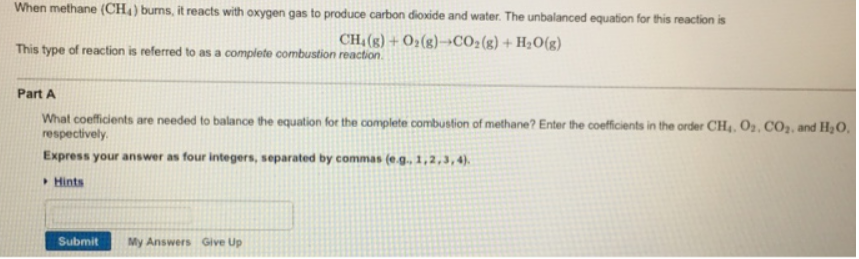# Problem: When methane (CH4) bums it reacts with oxygen gas to produce carbon dioxide and water. The unbalanced equation for this reaction is CH4 (g) + O2 (g) → CO2 (g) + H2O (g) This type of reaction is referred to as a complete combustion reaction. Part AWhat coefficients are needed to balance the equation for the complete combustion of methane? Enter the coefficients in the order CH4, O2, CO2, and H2O, respectively. Express your answer as four Integers, separated by commas (e g.. 1,2, 3, 4).

###### FREE Expert Solution
93% (247 ratings)###### Problem Details

When methane (CH4) bums it reacts with oxygen gas to produce carbon dioxide and water. The unbalanced equation for this reaction is

CH4 (g) + O2 (g) → CO2 (g) + H2O (g)

This type of reaction is referred to as a complete combustion reaction.

Part A

What coefficients are needed to balance the equation for the complete combustion of methane? Enter the coefficients in the order CH4, O2, CO2, and H2O, respectively.

Express your answer as four Integers, separated by commas (e g.. 1,2, 3, 4).